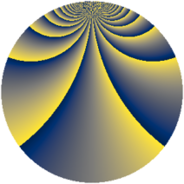# Properties

 Label 2760.2.yLevel $2760$ Weight $2$ Character orbit 2760.y Rep. character $\chi_{2760}(1979,\cdot)$ Character field $\Q$ Dimension $528$ Sturm bound $1152$

# Related objects

## Defining parameters

 Level: $$N$$ $$=$$ $$2760 = 2^{3} \cdot 3 \cdot 5 \cdot 23$$ Weight: $$k$$ $$=$$ $$2$$ Character orbit: $$[\chi]$$ $$=$$ 2760.y (of order $$2$$ and degree $$1$$) Character conductor: $$\operatorname{cond}(\chi)$$ $$=$$ $$120$$ Character field: $$\Q$$ Sturm bound: $$1152$$

## Dimensions

The following table gives the dimensions of various subspaces of $$M_{2}(2760, [\chi])$$.

Total New Old
Modular forms 584 528 56
Cusp forms 568 528 40
Eisenstein series 16 0 16

## Trace form

 $$528q + O(q^{10})$$ $$528q - 8q^{10} + 16q^{16} + 12q^{24} - 34q^{30} + 56q^{36} + 8q^{40} + 528q^{49} + 12q^{54} - 24q^{60} - 24q^{64} - 104q^{66} + 72q^{70} - 56q^{75} - 120q^{76} - 68q^{84} + 22q^{90} + 136q^{94} - 80q^{96} - 64q^{99} + O(q^{100})$$

## Decomposition of $$S_{2}^{\mathrm{new}}(2760, [\chi])$$ into newform subspaces

The newforms in this space have not yet been added to the LMFDB.

## Decomposition of $$S_{2}^{\mathrm{old}}(2760, [\chi])$$ into lower level spaces

$$S_{2}^{\mathrm{old}}(2760, [\chi]) \cong$$ $$S_{2}^{\mathrm{new}}(120, [\chi])$$$$^{\oplus 2}$$